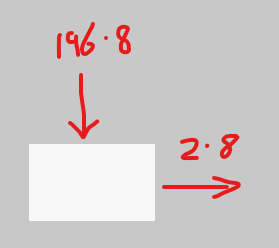# No good way to apply forces to part (Using physics formulas)

Hello, I have been trying to reliably apply forces on parts on Roblox, and I have found it pretty hard to do so, the problem I am having is that the simple formula F = ma does not work.

If you use a VectorForce object for example, let’s say part’s mass is 8 and the acceleration we want is 2, then F = 8 * 2, F = 16, if we use a Vector3.new(16, 0, 0) as a force on this part, the part won’t even move. I have tested many stuff and noticed that the friction changes the force necessary to move the part, making it even harder to understand what you have to do to get the force right.

Now let’s try AssemblyLinearVelocity, incrementing a velocity variable with force * dt / mass and setting this variable to the AssemblyLinearVelocity worked pretty well, except now all external forces won’t work, example: gravity.

What I would like is a good explanation of how I could tackle such problem, since Roblox has basically no documentation about its own physics system.

You are correct that friction affects the force necessary to move the part. If you want to accelerate a part along the ground you have to add enough force to counteract friction in addition to the force needed to achieve desired acceleration.

We can add the friction force to get this expanded formula:
`force = (mass * acceleration) + (gravity * mass * friction)`

This is assuming you are moving it along FLAT ground.

The default friction is 0.3 and default gravity is 196.2

``````force = 8 * 2 + 196.2 * 8 * 0.3
= 486.88
``````

Extra Notes:
You can use `workspace.Gravity` to make sure you always use the correct number for gravity.
If you are using custom physical properties, you must calculate the friction using the formula on this page:

2 Likes

I think the problem you have having is a problem of units and scale. 2 what? 16 what? Its arbitrary in a game somewhat.

The reason why your object won’t move is because it is also being acted on by game gravity which is much bigger than ‘2’. Look at your game gravity setting, its likely 196.2 ‘studs per second’. So you’re 2 * 8 is competing with a gravity force of 196.2 * 8 (a much larger number holding the object in place)Also, you can go into custom physical properties and reduce the friction to make a part slippery like ice.

Thanks for the help everyone, that worked perfectly, but another thing i cant get right is torque, lets say again i have a cylinder with radius 1 and mass 1, so inertia is 1, and the torque necessary to accelerate this cylinder at 1 stud per second would be 1, problem is that friction enters in the game again but at the inverse purpose, lowering the friction causes the wheel to not to accelerate, due to the formula, F = u * N, problem is that doubling the friction doesnt double the force, and 1 unit of torque doesnt accelerate the cylinder at 1 stud per second, to have that behavior, you need to multiply the torque by 1.45 (i dont know why, but i would like to)